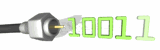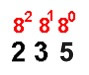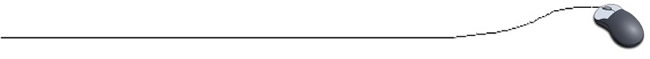Change a number from any base
back to base 10:The computer is representing a number typed at the keyboard by a pattern of ON and OFF signals represented by the binary 1011.  What number was typed at the keyboard to produce this pattern? Let's see how we can quickly convert numbers back to base 10 so that they will have more meaning to us humans.

Example 1:  Convert 2358 into base 10.The Process:
Above each of the digits in your number, list the power of the base that the digit represents.  See the example on the left.  It is now a simple process of multiplication and addition to determine your base 10 number.  In this example you have
 5 x 80 = 5   3 x 81 = 24 2 x 82 = 128 Now simply add these values together. 5 + 24 + 128 = 157 Answer: 2358 = 15710

** Remember: any number to the zero power equals one.Example 2:  Let's do the problem mentioned at the top of this lesson.
Convert 10112 to base 10.

The process is the same as in Example 1.

 1 x 20 = 1   1 x 21 = 2 0 x 22 = 0 1 x 23 = 8 1 + 2 + 0 + 8 = 11   Answer:  10112 = 1110Example 3:  Convert 1C416 to base 10.

 4 x 160 = 4   C x 161 = 12 x 161 = 192 1 x 162 = 256 4 + 192 + 256 = 452 Answer: 1C416 = 45210Notice: These materials are free for your on-line use at this site, but are not free for the taking. Please do not copy these materials or re-post them to the Internet, as it is copyright infringement. If you wish hard-copies of these materials, refer to our subscription area, JavaMathBits.com. Help us keep these resources free. Thank you.